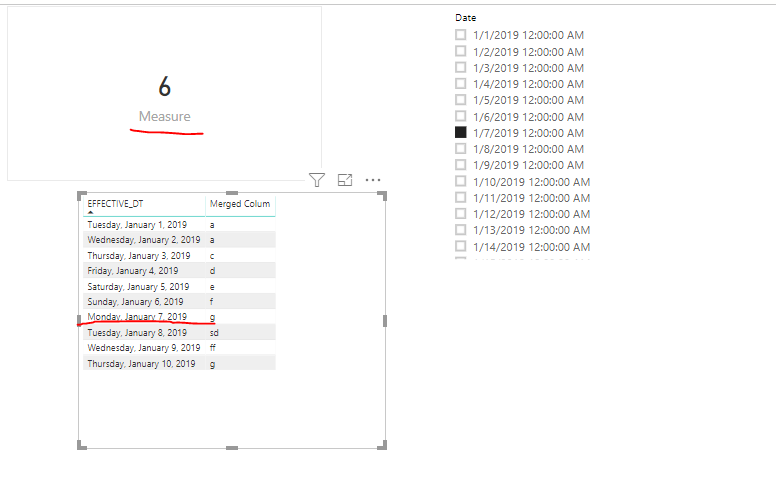cancel
Showing results for
Did you mean:Anonymous
Not applicable

## Dynamically calculate Measure value based on Slicer Selection

Hi,

My requirement is create measure which calculate distinct count of rows and i have one date column which i need to check with user selected Date slicer.

CONTROL PROVIDERS = CALCULATE(DISTINCTCOUNT(NETWORK[Merged Colum]),FILTER(NETWORK,NETWORK[EFFECTIVE_DT]<=SELECTEDVALUE('Date'[Start_Date])))

I Created one date table with start date column which has property "After".

SO my measure is showing blank values. I dont know why.
2 ACCEPTED SOLUTIONSCommunity Support

Hi @Anonymous ,

Did you create relationship between date table and the fact table in your scenario? Here I created a sample for your reference.

`Measure = CALCULATE(DISTINCTCOUNT(NETWORK[Merged Colum]),FILTER('NETWORK','NETWORK'[EFFECTIVE_DT]<=SELECTEDVALUE('Date'[Date])))`Pbix as attached.

Community Support Team _ Frank
If this post helps, then please consider Accept it as the solution to help the others find it more quickly.Super User

Hi,

Create a relationship from the EFFECTIVE_DT column to the Start Date column of the Date Table.  Create a slicer and drag Start Date column from the Date Table.  Select a Date there.  Write these measure

Count = DISTINCTCOUNT(NETWORK[Merged Colum])

CONTROL PROVIDERS = CALCULATE([Count],DATESBETWEEN('Date'[Start_Date],MINX(ALL(Date),'Date'[Start_Date]),MAX('Date'[Start_Date])))

Hope this helps.

Regards,
Ashish Mathur
http://www.ashishmathur.com
2 REPLIES 2Super User

Hi,

Create a relationship from the EFFECTIVE_DT column to the Start Date column of the Date Table.  Create a slicer and drag Start Date column from the Date Table.  Select a Date there.  Write these measure

Count = DISTINCTCOUNT(NETWORK[Merged Colum])

CONTROL PROVIDERS = CALCULATE([Count],DATESBETWEEN('Date'[Start_Date],MINX(ALL(Date),'Date'[Start_Date]),MAX('Date'[Start_Date])))

Hope this helps.

Regards,
Ashish Mathur
http://www.ashishmathur.comCommunity Support

Hi @Anonymous ,

Did you create relationship between date table and the fact table in your scenario? Here I created a sample for your reference.

`Measure = CALCULATE(DISTINCTCOUNT(NETWORK[Merged Colum]),FILTER('NETWORK','NETWORK'[EFFECTIVE_DT]<=SELECTEDVALUE('Date'[Date])))`Pbix as attached.

Community Support Team _ Frank
If this post helps, then please consider Accept it as the solution to help the others find it more quickly.